### Taylor Series (Linearization)

The Taylor series (linearization) method is the most commonly used method to estimate the covariance matrix of the regression coefficients for complex survey data. It is the default variance estimation method used by PROC SURVEYREG.

Use the notation described in the section Notation to denote the residuals from the linear regression as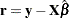with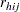as its elements. Let the
matrix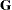be defined as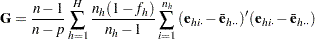where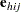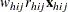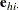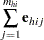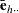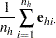The Taylor series estimate of the covariance matrix of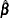is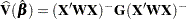The factor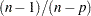in the computation of the matrixreduces the small sample bias associated with using the estimated function to calculate deviations (Hidiroglou, Fuller, and Hickman 1980). For simple random sampling, this factor contributes to the degrees of freedom correction applied to the residual mean square for ordinary least squares in whichparameters are estimated. By default, the procedure use this adjustment in the variance estimation. If you do not want to use this multiplier in variance estimation, you can specify the VADJUST=NONE option in the MODEL statement to suppress this factor.